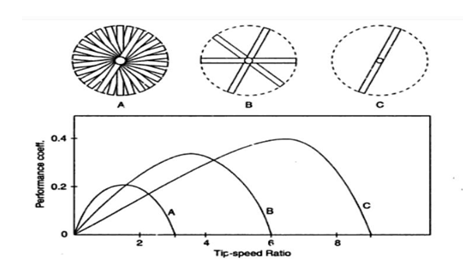Physics

# Presentation on TorqueTorque, τ , is tendency of a force to rotate object about some axis. Torque is vector quantity. Direction determined by axis of twist; perpendicular to both r and F, clockwise torques point into paper. Defined as negative; Counter-clockwise torques point out of paper. Defined as positive. Mathematically torque can be written as T = F * r * sin(theta), and it has units of Newton-meters.